Topic 5 min read# CVSim as a learning tool III: Linearity in EIS

Latest updated: January 5, 2022

CVSim is an extremely powerful access-free simulation tool in EC-Lab®, that allows anyone to understand cyclic voltammetry and its use in kinetic studies of redox reactions. It offers the possibility to simulate the response of a redox reaction to potential linear ramps but also to potential sine ramps. Consequently, CVSim can be used to study the behaviour of a redox reaction (E) for example, during an Electrochemical Impedance Spectroscopy (EIS) measurements.

## How to recognize a linear behavior during EIS experiment using Lissajous and/or Discrete Fourier Transform

The parameters used are shown in Fig. 1. The potential sine modulation and the current responses can be plotted as shown in Fig. 2. For an amplitude of 10 mV, the current response is also sinusoidal, after one period. The Lissajous plot, which is simply $I\,vs.\,E_\mathrm{WE}$ for a sinusoidal modulation, is shown in Fig. 3. Its elliptical shape is proof that the potential amplitude of 10 mV does not produce a non-linear response of the redox system.

Another way to check this is to plot the Discrete Fourier Transform (DFT) of the current response. This can be done using the Fourier Transform tool in EC-Lab® (Analysis->Math->Fourier Transform). The results are shown in Fig. 4. There is only one line at the frequency of the excitation 1 mHz, which means the system behaves like a linear system.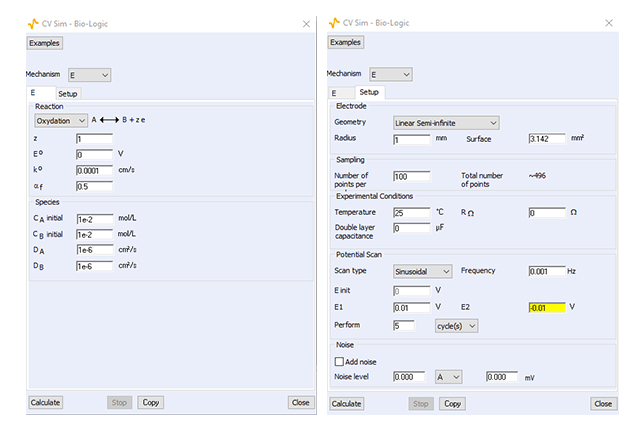Figure 1: Settings used for the simulation of the behavior of the (E) reaction during EIS measurements.
Both redox species are initially present in the solution.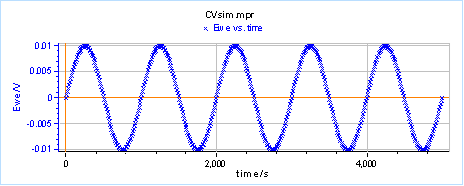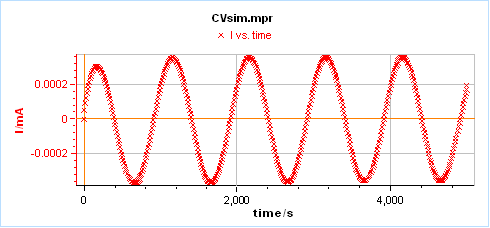Figure 2: Potential modulation and current response for the redox reaction (E) in the conditions shown in Fig. 1.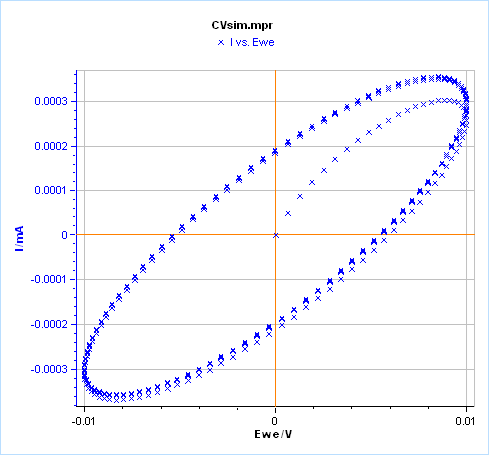Figure 3: Lissajous plot of the Fig. 2.Figure 4: Fourier Transform of the current response shown in Fig. 2.

If we use a potential modulation of 50  mV by setting E1 and E2 equal to 0.05 and -0.05 V, respectively, we can see that the current response is totally different (Fig. 5). The current response does not look like a single sine wave anymore and seems to be distorted. The Lissajous plot looks less elliptical than before (Fig. 6) even after the first period of the signal.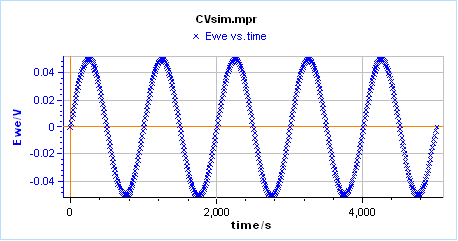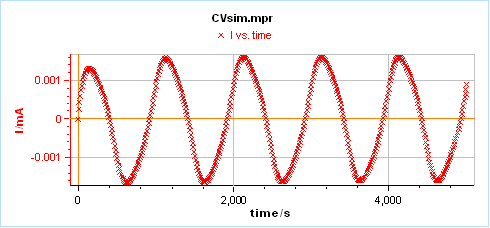Figure 5: Potential and current response for the redox reaction (E) in the conditions shown in Fig. 1
except for the input potential modulation amplitude, which is equal to 50 mV instead of 10 mV.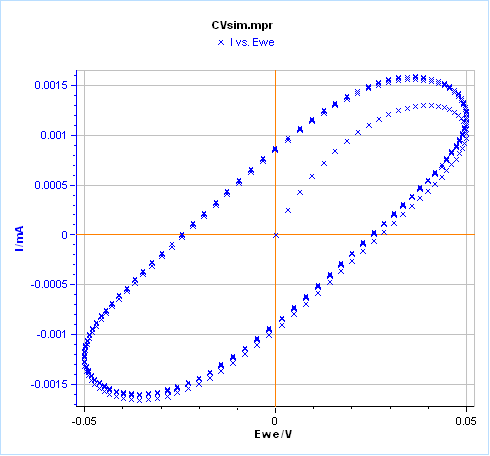Figure 6: Lissajous plot of the Fig. 5.

Finally, if we plot the DFT of the current response (Fig. 7), we have two lines, one at the excitation frequency f and another at the frequency 3f, which is the definite proof that the system does not behave linearly. The fact that there is only the 3rd harmonic and not the 2nd harmonic is explained by the choice of a symmetry factor αf=0.5. If we choose a symmetry factor αf=0.1, the second harmonic starts to show up (Fig. 8). We would see even more harmonics if we could increase the amplitude of the signal.

This topic also shows how using the FT of the signal to assess non-linearity is much more sensitive and convenient than using the Lissajous plot, for which the discrimination parameter ( ie the shape of the plot) is less quantitative.

The DFT is used to calculate the quality indicators [1,4].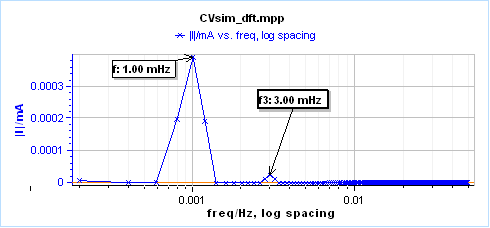Figure 7: Fourier Transform of the current response shown in Fig. 5. Additional signal can be seen at 3 mHz.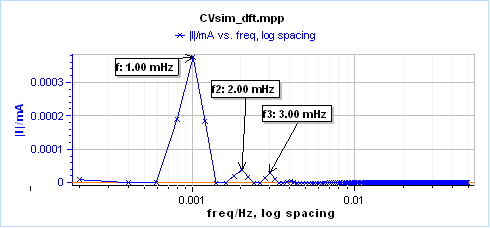Figure 8: Fourier Transform using the same parameters as in Fig. 7 but with a symmetry factor
$\alpha_\text{f}$ equal to 0.1. Additional signal can be seen at 2 and 3 mHz.

CVSim Electrochemical Impedance Spectroscopy EIS Lissajous Discrete Fourier Transform Linearity Discrete Fourier Transform Linearity

The following articles, in the same series, may be of interest...

## Related products

The following products may be of interest...

## Related accessories

The following accessories may be of interest...

Work smarter. Not harder.

Tech-tips, theory, latest functionality, new products & more.

No thanks!